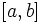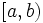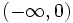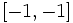# Space of Riemannian metrics

## Definition

### Given data

A differential manifold$M$.

### Definition part

The space of Riemannian metrics on$M$ is the set of all Riemannian metrics on$M$. Since each Riemannian metric can be viewed as a$(0,2)$-tensor (viz a section of the$(0,2)$-tensor bundle), the space of Riemannian metrics can be viewed as a subset of the space of all sections of the$(0,2)$-tensor bundle.

The space of Riemannian metrics is studied as a topological space, with the smooth topology.

The space of Riemannian metrics is important because we are interested in studying evolutions of Riemannian metrics under flow equations, and the manifold over which these flow equations are considered is precisely the space of Riemannian metrics.

## Topological properties

### Contractibility

The space of Riemannian metrics of a differential manifold is contractible. This means, in particular, that all its homotopy groups, and homology groups, are trivial.

## Subspaces

### Subspaces obtained by curvature bounds

Given two (extended) real numbers$a$ and$b$, we can consider the subset of the space of Riemannian metrics comprising those Riemannian metrics for which the sectional curvature everywhere lies in$[a,b]$ or$[a,b)$ or$(a,b]$ or$(a,b)$. Some particular examples are:

•$(-\infty,0)$ describes the space of negatively curved metrics
•$(-\infty,0]$ describes the space of nonpositively curved metrics
•$[-1,-1]$ describes the space of hyperbolic metrics

The Ricci flow on surfaces actually shows that for a surface, the space of hyperbolic metrics is a deformation retract of the space of negatively curved metrics.

## Quotients and moduli

### Riemannian metrics upto conformal equivalence

We declare two Riemannian metrics to be conformally equivalent if there is a scalar, everywhere nonzero function that multiplied with one Riemannian metric, gives the other. This is essentially equivalence under the action of the multiplicative group of everywhere positive functions, on the tensor bundle. The quotient of the space of Riemannian metrics under the equivalence relation of conformal equivalence, is termed the space of conformal classes of Riemannian metrics.

### Riemannian metrics upto diffeomorphism equivalence

We declare two Riemannian metrics to be diffeomorphically equivalent if there is a diffeomorphism of the underlying manifold that takes one Riemannian metric to the other. Here, we use the fact that when there is a local diffeomorphism, we can pull back Riemannian metrics in a unique way. The moduli space obtaiend by quotienting out by this equivalence relation is termed the space of Riemannian metrics up to diffeomorphism.

## Points in the space of Riemannian metrics

The question: given a differential manifold, can it be equipped with a metric having a given property? translates to the question: given a differential manifold, is there a point in its space of Riemannian metrics that satisfies the given property?

To prove that there does exist a point satisfying the given property, one of the tools we use is to consider a differential operator on the space of Riemannian metrics such that the Riemannian metrics it maps to zero are precisely those having the property. We then look at the flow equation for this differential operator, and try to find a trajectory for the flow equation that converges at$\infty$ or$-\infty$.

Further information: Flow method to prove existence of certain metrics